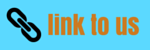# Thermodynamics

## 5. Internal Energy and first law of thermodynamics

• Internal energy can be described as the sum of kinetic and potantial energies of individual movecules in the material.
• But in thermodynamics one should keep in mind that U is simply a macrosopic variable of the system.
• U is thermodynamic state variable and its value depends only on the given state of the system and not on path taken to arrive the state.
• Transfer of heat and performance of work are two mean of adding or subtracting energy from a system.
• On transfer of energy, system is said to have undergone a change in internal energy.
• Thus the sum of heat put into the system plus work done on the system equals increase in internal energy of the system for any process.
if, U1 is internal energy of state 1 and U2 is internal energy of state 2 than change in internal energy would be
ΔU=U2 - U1
• If W is the work done by the system on its surroundings then -W would be the work done on the system by the surroundings .
• If Q is the heat put into the system then,
Q+(-W)=ΔU
usually written as
Q=ΔU+W                     (4)
• Equation (4) is then know as first law of thermodynamics and it can be applied when
Q, W and U are expressed in same units.
Some Imp stuff      (1) Q is positive when heat is given to the system and Q is negative when heat is taken from the system
(2) W is positive when system expands and does work on surroundings
• Hence we may say that when a certain amount of heat Q is given to the system then some part of it is used in increasing internal energy ΔU of the system while remaining part leaves the system in form of work done by the system on its surroundings.
• From equation 4 we see that first law of thermodynamics is a statement of conservation of energy stated as
' The energy put into the system equals the sum of the work done by the system and the change in internal energy of the system'
• If the system undergoes any process in which ΔU=0 i.e., charge in internal energy is zero then from (4)
Q = W
that is heat supplied to the system is used up enterely in doing work on the surroundings.

## 6. Specific heat capacity of an ideal gas

• We have defined specific heat capacity and molar specific heat capacity earlier in the previous chapter.
• There are two specific heats of ideal gases.
(i) Specific heat capacity at constant volume
(ii) Specific heat capacity at constant pressure
Cp and Cv are molar specific heat capacities of ideal gas at constant pressure and volume respectively for Cp and Cv of ideal gas there is a simple relation.
Cp-Cv = R                         (7)
where R- universal gascontant
• This relation can be proved as follows.
from first law of thermaodynamics for 1 mole of gas we have
ΔQ =Δ U+PΔV                    (8)
• If heat is absorbed at constant volume then ΔV = 0 and
CV=(ΔQ/ΔT)V=(ΔU/ΔT)V     (9)
If Q in absorbed at constant pressure than
CP=(ΔQ/ΔT)P=(ΔU/ΔT)P+P(ΔV/ΔT)P
now ideal gas equation for 1 mole of gas is
PV = RT
= P(ΔV/ΔT) = R                    (10)
from (9) and (10)
CP - CV=(ΔU/ΔT)P-(ΔU/ΔT)V+P(ΔV/ΔT)P
• Since internal energy U of ideal gas depands only on temperature so subscripts P and V have no meaning.
=>          CP - CV = R
which is the desired relation